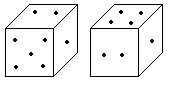# Verbal Reasoning - Dice - Discussion

### Discussion :: Dice - Dice 1 (Q.No.2)

2.

Two positions of dice are shown below. How many points will appear on the opposite to the face containing 5 points?[A]. 3 [B]. 1 [C]. 2 [D]. 4

Explanation:

In these two positions one of the common face having 1 point is in the same position. Therefore according to rule (2). There will be 4 points on the required face.

 Harshit said: (Dec 1, 2013) Didn't get how it's solved. Please tell what is rule 2?

 Pawan said: (Jun 11, 2014) It is not cleared that how to follow rule 2 and not justified the answer how to get 4?

 Abhishek Vimal said: (Oct 24, 2014) Tell me in detail how the answer 4 comes?

 Siva Raman said: (Nov 10, 2014) If face of 2 points is rotated to down, 5 points will go to bottom face, now 2 is in center and 4 is upper face. Hence 4 is opposite to 5.

 Ajish said: (Dec 4, 2014) In such question if 2 faces are common, remaining uncommon will be opposite to each other. It is proven. If you have doubt kindly search DSC'S Dice Lecture in you tube. All the best.

 Pravin said: (Mar 1, 2015) Explain me details?

 Pravin said: (Mar 1, 2015) Explain me details?

 Deepak Allu said: (Mar 5, 2015) ANS : D. Consider the dice in figure 1. Hold the dice shown in figure in your hand such that the side having 5 points is facing you. Then on your right (adjacent to 5) we have 1, and the top side will be 2. If you rotate the dice such that the side having 2 points is facing you, then we get the second figure. Hence the answer is 4.

 Keerthana said: (Mar 11, 2015) The question is opposite to face 5 points. Lets see the both dices in points = 5, 2, 1 and 4, 2, 1. (all are nearest points right). 2 and 1 is common for both the dices. 4 only come to opposite side and so on 2 and 1 are already near to 5. Hence 4 is the answer.

 Dorjay said: (Aug 4, 2015) Earlier I was bit too confused about rule 2 now I understand. It says If there are two common faces then and one is at common position then remaining faces (two faces left) that will be opposite to each other. i.e In position 1 there are three faces 2 5 1. In position 2 there are three faces 4 2 1. Two common faces (1 at common position and 2 not at common position) exactly fit in rule 2 description. So the remaining left is 5 and 4 they will be opposite to each other. Total six faces in two position (3+3) = (1 2) & 5 + (1 2) & 4 common. So 5 and 4 left they are opposite. Only difference between rule 2 & rule 3 is that in rule 3 only one faces is common and that is at common position.

 Sateesh said: (Jan 8, 2016) Very simple: Just strike the common numbers (2, 1) of both dice, then remaining numbers on the both dice are 5-4.

 Shahid said: (Mar 19, 2016) How we can learn this kind of question I am trying to solve this kind of question, however this goes out of mind so please give me any suggestions.

 Pk(Praveenkumar) said: (Apr 28, 2016) What is rule no.2? Explain the rule.

 Surinder Kumar said: (Oct 4, 2016) Please tell me rule 2.

 D!P said: (Mar 23, 2017) 1 is same direction of two dice. So 2 ----- 4 or 2 ---- 5.

 Veerpal Kaur said: (May 16, 2017) Here the sum of any two side of side is 7 so it is general dice and in case of general dice we have. 1 opposite to 6. 2 opposite to 5. 3 opposite to 4? But it is following the rule of standard dice how? Aan anyone explain?

 Sahil Indorkar said: (Jul 26, 2017) According to rule, when two faces of each dice are common. Another face are always common. In 1st dice (2, 1 are appeared). In 2nd dice (2, 1 are appeared). Remaining are 5 and 4 so according to the rule, they are opposite to each other.

 Renu Adhikari said: (Apr 22, 2018) How will answer 4 while 5 point side face is 2 point? Please explain.

 Smruti said: (May 24, 2018) According to the rule, In this case, if the two numbers are common in both the dices then the left number of 1st dice is opposite to the left number of 2nd dice. In 1st and 2nd dice 2&1appear. And rest 5&4so they are opposite to each other.

 Aryadrj said: (Feb 12, 2019) The common number will be canceled and the rest number will be opposite to each other. 11 and 22 will be cancel and 4 and 5 will be common.

 Rahul Kumar said: (Mar 5, 2019) @All. If you will rotate the first dice to make the position of no 2 = no 2 of the second dice the opposite face of no 5 will be 4.

 Sanghamitra said: (Mar 17, 2019) Actually, there is a rule if two faces are common then pair that common two faces and remaining faces will opportunities to each other. In this case, there are two common faces in two dice that means in 1st dice there is 1 and 2 and in second dice there is 1 and 2 then in 1st dice 5 remain and in second dice 4 remain so opposite of 5 is 4.

 Bikram said: (May 15, 2019) It's wrong because one side of dice is general while other is standard, one dice can't be general and standard both.